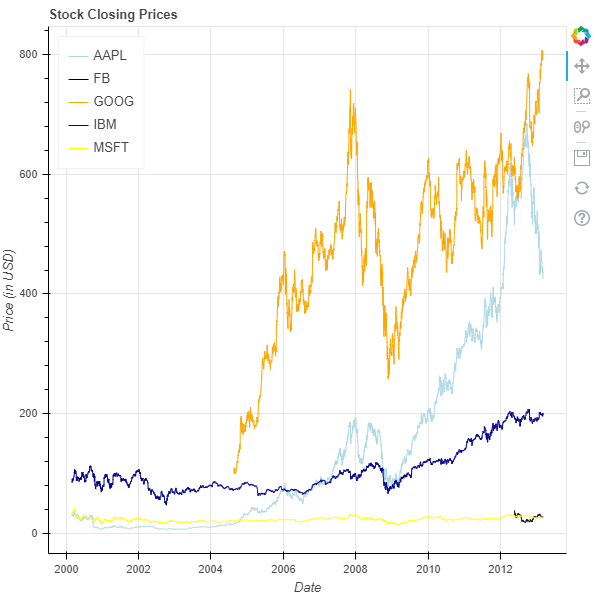GFG App
Open AppBrowser
Continue

# Python Bokeh – Visualizing Stock Data

Bokeh is a Python interactive data visualization. It renders its plots using HTML and JavaScript. It targets modern web browsers for presentation providing elegant, concise construction of novel graphics with high-performance interactivity.
Bokeh can be used to visualize stock market data. Visualization is be done using the `plotting` module. Here we will be using the sample stock datasets given to us by Bokeh.

To download the sample datasets run the following command on the command line :

`bokeh sampledata`

Alternatively, we can also execute the following Python code :

```import bokeh
```

## Analyzing the dataset :

In the sample data provided by Bokeh, there are datasets of the stocks of the following companies :

• AAPL which is Apple
• IBM which is International Business Machines
• MSFT which is Microsoft Corporation

All these datasets are available as CSV files. Below is a glimpse into the IBM.csv file :

```Date        Open    High    Low    Close    Volume        Adj Close
01-03-2000    102    105.5    100.06    100.25    10807800    84.48
02-03-2000    100.5    105.44    99.5    103.12    11192900    86.9
03-03-2000    107.25    110    106.06    108    10162800    91.01
06-03-2000    109.94    111    101    103.06    10747400    86.85
07-03-2000    106    107    101.69    103    10035100    86.8
```

The file contains the stock data between the years 2000 and 2013 with over 3000 entries.

## Visualizing the Stocks :

We will be plotting a line graph which will track the closing price of the stocks between the years 2000 and 2013 of all the 5 available companies.

1. Import the required modules :
• numpy
• figure, output_file and show from bokeh.plotting
• AAPL, FB, GOOG, IBM and MSFT from bokeh.sampledata.stocks
2. Instantiate a figure object with the title and axis types.
3. Give the names to x-axis and y-axis.
4. Plot line graphs for all the 5 companies.
5. Display the model.

 `# importing the modules ` `import` `numpy as np ` `from` `bokeh.plotting ``import` `figure, output_file, show ` `from` `bokeh.sampledata.stocks ``import` `AAPL, FB, GOOG, IBM, MSFT ` `  `  `# the file to save the model ` `output_file(``"gfg.html"``) ` `  `  `# instantiating the figure object ` `graph ``=` `figure(x_axis_type ``=` `"datetime"``, title ``=` `"Stock Closing Prices"``) ` `  `  `# name of the x-axis ` `graph.xaxis.axis_label ``=` `'Date'` `  `  `# name of the y-axis ` `graph.yaxis.axis_label ``=` `'Price (in USD)'` `  `  `# plotting the line graph for AAPL ` `x_axis_coordinates ``=` `np.array(AAPL[``'date'``], dtype ``=` `np.datetime64) ` `y_axis_coordinates ``=` `AAPL[``'adj_close'``] ` `color ``=` `"lightblue"` `legend_label ``=` `'AAPL'` `graph.line(x_axis_coordinates, ` `        ``y_axis_coordinates, ` `        ``color ``=` `color, ` `        ``legend_label ``=` `legend_label) ` `  `  `# plotting the line graph for FB ` `x_axis_coordinates ``=` `np.array(FB[``'date'``], dtype ``=` `np.datetime64) ` `y_axis_coordinates ``=` `FB[``'adj_close'``] ` `color ``=` `"black"` `legend_label ``=` `'FB'` `graph.line(x_axis_coordinates, ` `        ``y_axis_coordinates, ` `        ``color ``=` `color, ` `        ``legend_label ``=` `legend_label) ` `  `  `# plotting the line graph for GOOG ` `x_axis_coordinates ``=` `np.array(GOOG[``'date'``], dtype ``=` `np.datetime64) ` `y_axis_coordinates ``=` `GOOG[``'adj_close'``] ` `color ``=` `"orange"` `legend_label ``=` `'GOOG'` `graph.line(x_axis_coordinates, ` `        ``y_axis_coordinates, ` `        ``color ``=` `color, ` `        ``legend_label ``=` `legend_label) ` `  `  `# plotting the line graph for IBM ` `x_axis_coordinates ``=` `np.array(IBM[``'date'``], dtype ``=` `np.datetime64) ` `y_axis_coordinates ``=` `IBM[``'adj_close'``] ` `color ``=` `"darkblue"` `legend_label ``=` `'IBM'` `graph.line(x_axis_coordinates, ` `        ``y_axis_coordinates, ` `        ``color ``=` `color, ` `        ``legend_label ``=` `legend_label) ` `  `  `# plotting the line graph for MSFT ` `x_axis_coordinates ``=` `np.array(MSFT[``'date'``], dtype ``=` `np.datetime64) ` `y_axis_coordinates ``=` `MSFT[``'adj_close'``] ` `color ``=` `"yellow"` `legend_label ``=` `'MSFT'` `graph.line(x_axis_coordinates, ` `        ``y_axis_coordinates, ` `        ``color ``=` `color, ` `        ``legend_label ``=` `legend_label) ` `  `  `# relocating the legend table to  ` `# avoid abstruction of the graph ` `graph.legend.location ``=` `"top_left"` `  `  `# displaying the model ` `show(graph) `

Output :My Personal Notes arrow_drop_up